# Let X = the time (in 10-1 weeks) from shipment of a defective product until the customer returns the product. Suppose that the minimum return time is y = 5.5 and that the excess X - 5.5 over the minimum has a Weibull distribution with parameters a = 2 and B = 1.5.(a) What is the cdf of X?x< 5.5F(x) = {x2 5.5(b) What are the expected return time and variance of return time? [Hint: First obtain E(X - 5.5) and V(X - 5.5).] (Round your answers to three decimal places.)E(X) =V(X) =|10-1 weeks(10-1 weeks)?(c) Compute P(X > 7). (Round your answer to four decimal places.)(d) Compute P(7 SXS 8.5). (Round your answer to four decimal places.)

Question
6 views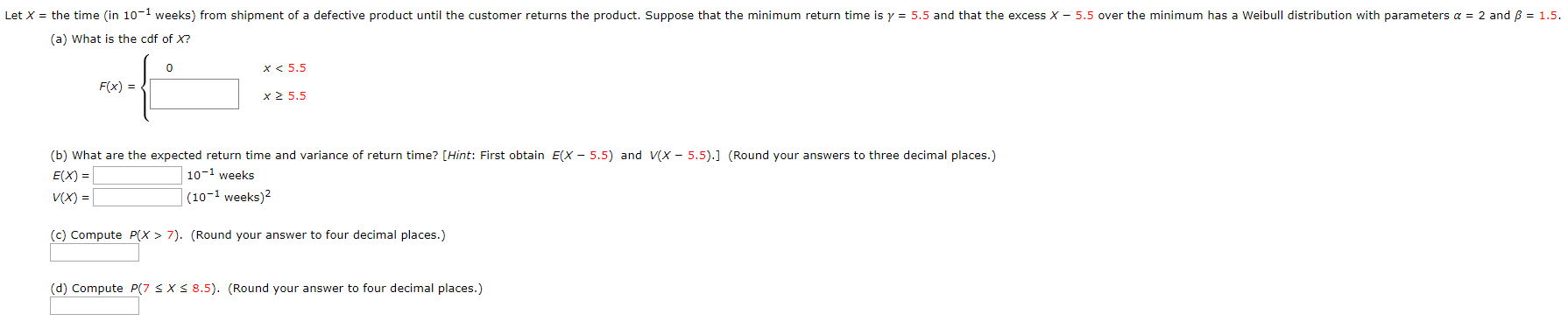help_outlineImage TranscriptioncloseLet X = the time (in 10-1 weeks) from shipment of a defective product until the customer returns the product. Suppose that the minimum return time is y = 5.5 and that the excess X - 5.5 over the minimum has a Weibull distribution with parameters a = 2 and B = 1.5. (a) What is the cdf of X? x< 5.5 F(x) = { x2 5.5 (b) What are the expected return time and variance of return time? [Hint: First obtain E(X - 5.5) and V(X - 5.5).] (Round your answers to three decimal places.) E(X) = V(X) =| 10-1 weeks (10-1 weeks)? (c) Compute P(X > 7). (Round your answer to four decimal places.) (d) Compute P(7 SXS 8.5). (Round your answer to four decimal places.) fullscreen
check_circle

Step 1

Note:

Hey there! Thank you for the question. As you have posted a question with more than 3 sub-parts, as per our policy, we have solved the first 3 sub-parts for you. If you need help with the other one, please re-post the question and mention it.

Step 2

(a)

The random variable (X ­– 5.5) is a 2-parameter Weibull distribution, with parameters α = 2, and β = 1.5. Thus, the random variable X is a 3-parameter Weibull distribution, with parameters α = 2, β = 1.5, and γ = 5.5.

The probability distribution function (pdf) and cumulative distribution function (cdf) of this distribution are given below: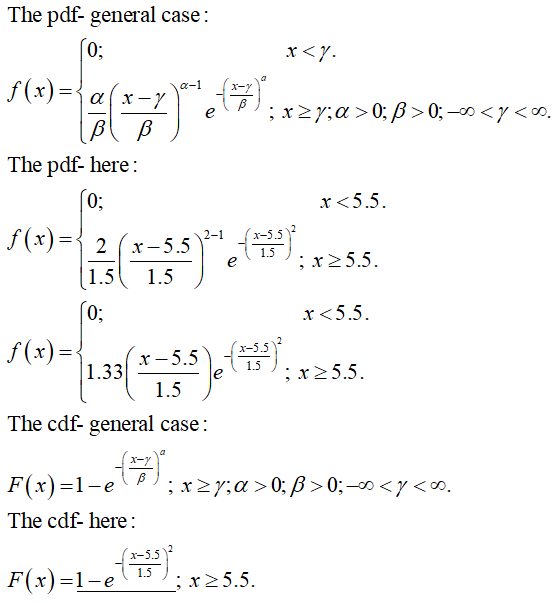Step 3

(b)

The expectation and variance for the 2-parameter Weibull distribution are: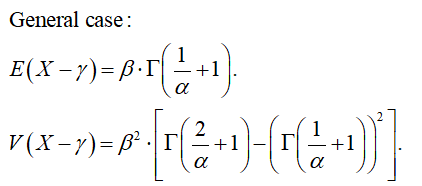Calculation: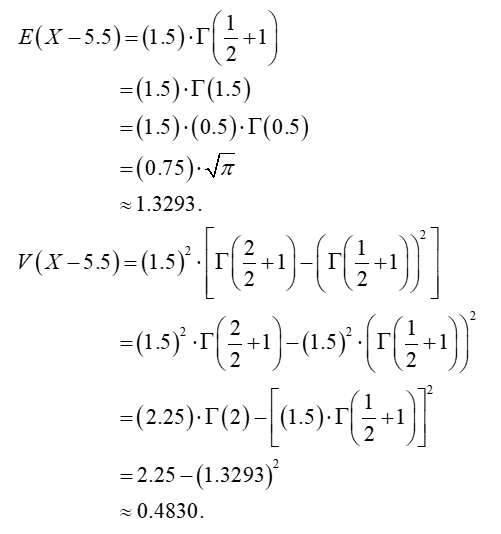Thus, the expectation and variance for the 3-parameter Weibull distribution are: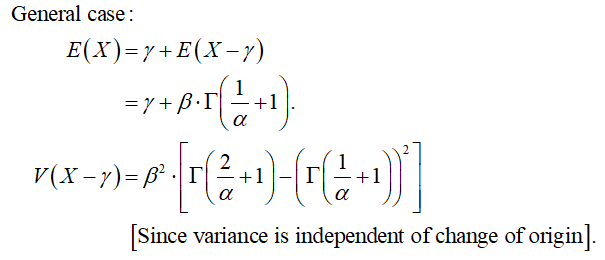Calculation: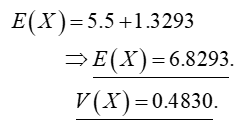...

### Want to see the full answer?

See Solution

#### Want to see this answer and more?

Solutions are written by subject experts who are available 24/7. Questions are typically answered within 1 hour.*

See Solution
*Response times may vary by subject and question.
Tagged in